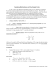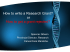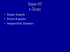# Document 6537334

## Transcription

Document 6537334
```STAT 352: Section 6.5 and 6.7: Large Sample Tests for the difference of two means and Small Sample Tests
for the difference of two means: independent samples
Independent and dependent samples
Two samples are independent if the sample values selected from one population are not related to or somehow
paired or matched with the sample values selected from the other population.
Two samples are dependent (or consist of matched pairs) if the members of one sample can be used to
determine the members of the other sample.
COMPARING MEANS: INDEPENDENT SAMPLES
ST
FRAMEWORK: 1
nd
sample: x1, x2, …, xm from population with mean μx;
2 sample: y1, y2, …, y from population with mean μy;
n
GOAL: Determine if μx –μy =>< 0 or if μx –μy =>< ∆
Test Ho: μx – μy=≥≤ ∆0 vs Ha: μx - μy ≠ <>∆0; ∆0 is the difference b/w two means we want to test for.
COMPARING MEANS:
CASE 1: Large samples or
STEP1. Ho: μx – μy=≥≤ ∆0
STEP 2. Test statistic:
known
vs
𝑧=
known.
Ha: μx - μy ≠ ><∆0, significance level α.
(𝑥̅ −𝑦�)−∆0
𝜎 2
𝜎 2
� 𝑥 + 𝑦
𝑛𝑥
𝑛𝑦
Under the Ho, the test statistic has standard normal distribution.
STEP 3. Critical value? For one-sided test z , for two-sided z
α
α/2
.
STEP 4. DECISION-critical/rejection region(s) depends on Ha.
Ha: μx - μy ≠ ∆0 Reject Ho if |z|> z ;
α/2
Ha: μx - μy > ∆0 Reject Ho if z > z ;
α
Ha: μx - μy < ∆0 Reject Ho if z < - z .
α
STEP 5. Answer the question in the problem.
Note: If σx and σy are not known, but samples are large, substitute sx and sy in the formula for the test
statistic. Use also when small sample, but normal population and
COMPARING MEANS: σx and σy not known, normal populations.
CASE 2: σx and σy not known, but assumed equal.
STEP 2. Test statistic:
(𝑥̅ − 𝑦�) − ∆
𝑡 =
1
1
𝑠𝑝 �𝑛 + 𝑛
𝑥
𝑦
where
𝑠𝑝2 is a pooled estimate of the common variance
Under the Ho, the test statistic has t distribution with df = m+n-2.
known.
STEP 3. Critical value? One-sided test tα, two-sided tα/2 .
STEP 4. DECISION-critical/rejection region(s) depends on Ha.
Ha: μx - μy ≠ ∆0 Reject Ho if |t|> tα/2;
Ha: μx - μy > ∆0 Reject Ho if t > tα;
Ha: μx - μy < ∆0 Reject Ho if t < - tα.
COMPARING MEANS: σx and σy not known, normal populations.
CASE 3: σx and σy not known, and may not be assumed equal.
STEP 2. Test statistic:
(𝑥̅ − 𝑦�) − ∆
𝑡 =
𝑠 2 𝑠 2
� 𝑥 + 𝑦
𝑛𝑥
𝑛𝑦
Under Ho, the degrees of freedom for the t distribution may be approximated by
STEP 3. Critical value? One-sided test t , two-sided t
α
α/2
.
STEP 4. DECISION-identical to CASE 2
EXAMPLES:
1. A medication for blood pressure was administered to a group of 13 randomly selected patients with elevated
blood pressure while a group of 15 was given a placebo. At the end of 3 months, the following data was
obtained on their Systolic Blood Pressure.
Control group, x: n=15, sample mean = 180, s=50
Treated group, y: m=13, sample mean =150, s=30.
Test if the treatment has been effective. Assume the variances are the same in both groups and use α=0.01.
Construct a 95% CI for the difference in the means of blood pressures for the two groups (μx - μy).
2. Sample statistics are shown for the distances of the home runs hit in record-setting seasons by Mark
McGwire and Barry Bonds. Use a 0.05 significance level to test the claim that the distances come from
populations with different means. McGwire: n=70,
Bonds: n=73,
=418.5, and s = 45.5
=403.7, and s = 30.6
3. Good website design can make Web navigation easier. An article presents a comparison of item recognition
between two designs. A sample of 10 users using a conventional Web design averaged 32.3 items identified,
with a standard deviation of 8.56. A sample of 10 users using a new structured Web design averaged 44.1 items
identified, with a standard deviation of 10.09. Can we conclude that the mean number of items identified is
greater with the new structured design?
```

### HYPOTHESIS TESTING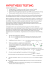### sonia cordero cardenas san andres university – la paz •jaime rojas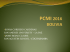### Stat 100 Sample Final Questions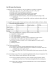### Review for the Final Exam (STA248)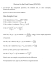### Population vs. Sample - Ms. Tomas` Math Page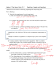### Chapter 11 and 12 Review 2015 ANSWERS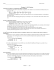### One-Sample Hypothesis Tests Review Problems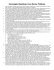### Inferential Statistics t and ScWk 242 – Session 9 Slides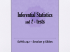### Hypothesis Testing Series (One Sample) STA2023-4a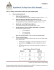### File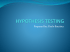### Document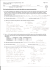### chapter 9 practice - faculty.piercecollege.edu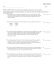### Job Search Form - Service Canada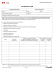### Elementary Statistics Sample Exam #3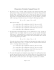### Review for Midterm II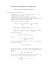### August 2012 EXAMINATIONS Solution – Part I (B)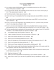### IntegraScreen Energy-efficient sorter for the best screening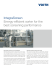### STAT 2000 Sample Midterm (actually given in October 2007)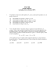### Chapter 7 Lecture Notes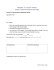### Sampling Distributions and One Sample Tests